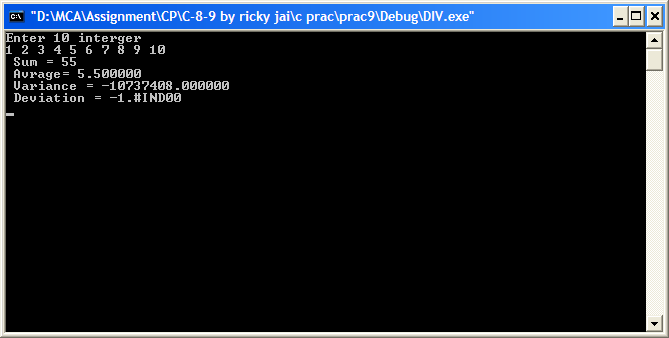## C program to add, average and deviation of numbers, C/C++ Programming

Assignment Help:

C program to add, average and deviation of numbers:

void main()

{

int sum=0,a,i;

float avg=0,dev,vari=0,var;

printf("Enter 10 interger \n");

for(i=0;i<10;i++)

{

scanf("%d",&a[i]);

sum=sum+a[i];

}

avg=sum*0.1;

for(i=0;i<10;i++)

var=var+pow((a[i]-avg),2);

vari=var/10;

dev=sqrt(vari);

printf(" Sum = %d \n Avrage= %f \n Variance = %f \n Deviation = %f \n",sum,avg,vari,dev);

getch();

}#### Area under curve, Write a program to find the area under the curve y = f(x)...

Write a program to find the area under the curve y = f(x) between x = a and x = b, integrate y = f(x) between the limits of a and b. The area under a curve betw

#### Simple object-oriented program, This assignment is to be undertaken individ...

This assignment is to be undertaken individually - no group work is permitted. Background information This assignment is an exercise in simple object-oriented programming and, acco

#### C program for total & average in the array , C Program for TOTAL  & AVERA...

C Program for TOTAL  & AVERAGE IN THE ARRAY  #include stdio.h> #include conio.h> #include string.h> void main() {           int i=0,n,limit=0,a=0;

#### Algorithm, Write algorithms to implement the insertion and deletion operati...

Write algorithms to implement the insertion and deletion operations.

#### Programing, #question. write a program number to alphabet in c++..

#question. write a program number to alphabet in c++..

#### What is abstraction?, What is abstraction? - Simplified view of an obje...

What is abstraction? - Simplified view of an object in user's language is termed as abstraction. - It's the simplest, well-defined interface to an object in OO and C++ which

#### C program to replace every occurrence of in a string, Program is to replace...

Program is to replace every occurrence of in a string: Program is to replace every occurrence of c1 in string with c2 and have function return the no. of replacement int re

#### How do i develop a subscript operator for a matrix class?, Employ operator ...

Employ operator () instead of operator[]. While you have multiple subscripts, the cleanest way to do it is along with operator () instead of with operator[]. The reason is that

#### Fundamental programing, Ask question #Minimum 100 words pted#acce

Ask question #Minimum 100 words pted#acce

#### #title areaundercurve.c, Write a program to find the area under the curve y...

Write a program to find the area under the curve y = f(x) between x = a and x = b, integrate y = f(x) between the limits of a and b. The area under a curve between two points can b